# Current Electricity Current Electricity Current Electricity is due

• Slides: 27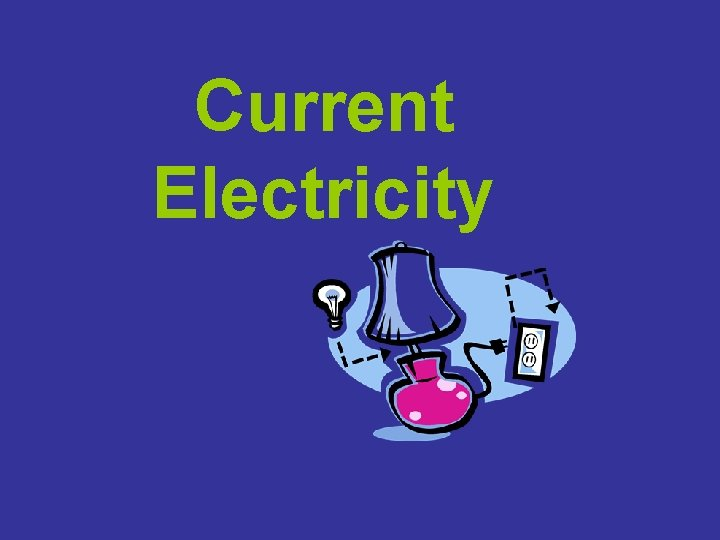Current Electricity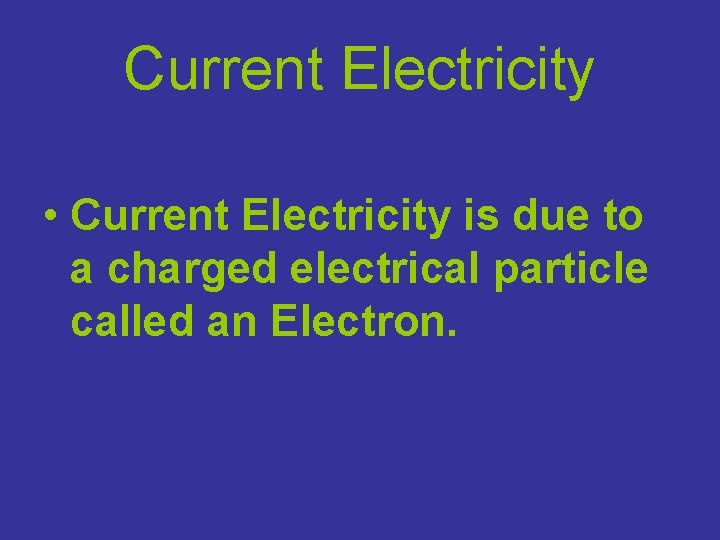Current Electricity • Current Electricity is due to a charged electrical particle called an Electron.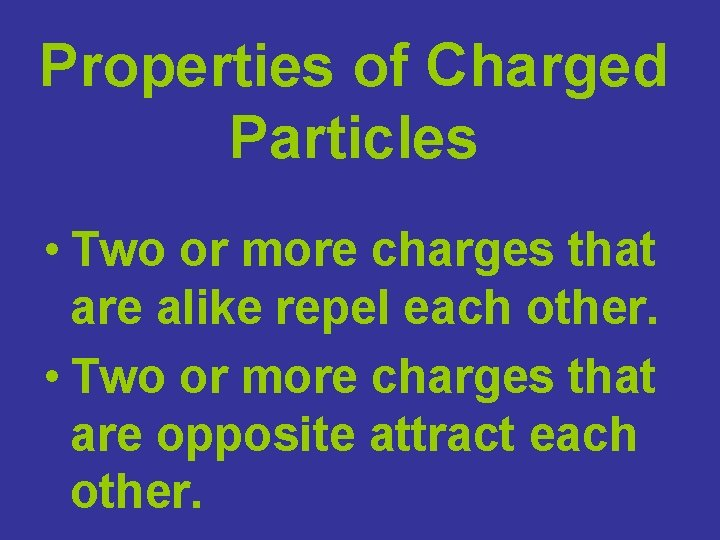Properties of Charged Particles • Two or more charges that are alike repel each other. • Two or more charges that are opposite attract each other.Current • The charge pump creates the FLOW of charged particles. • This is called a CURRENT.• Current is symbolized by the letter I. • It is measured in amperes, A.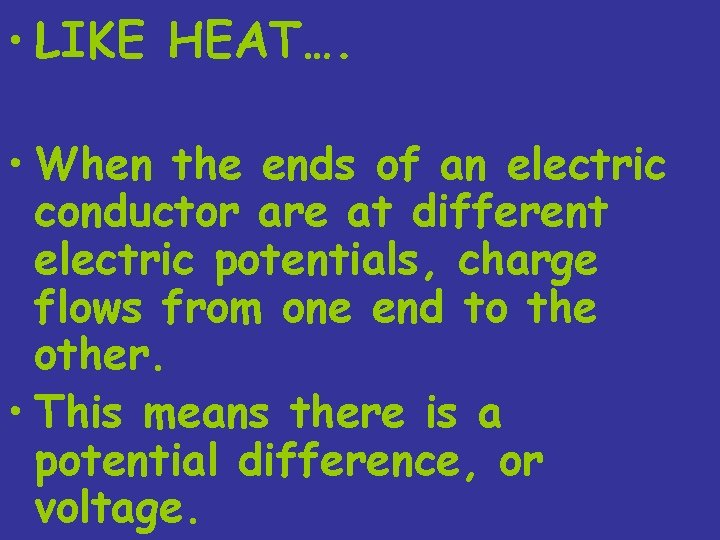• LIKE HEAT…. • When the ends of an electric conductor are at different electric potentials, charge flows from one end to the other. • This means there is a potential difference, or voltage.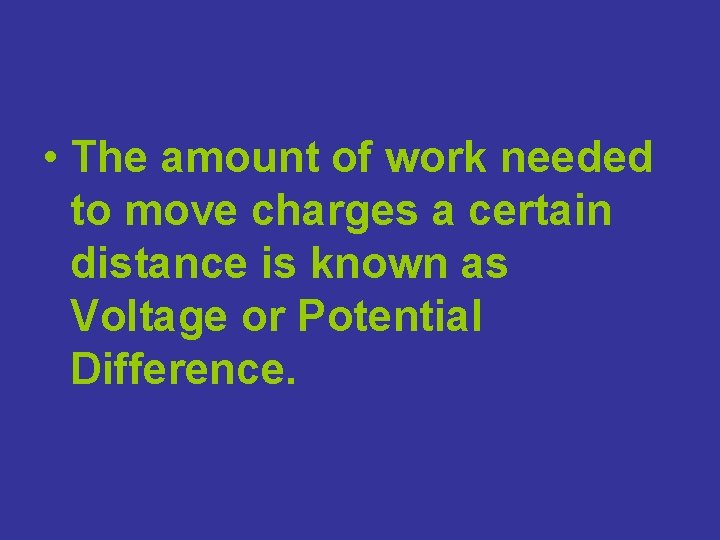• The amount of work needed to move charges a certain distance is known as Voltage or Potential Difference.Voltage • A measure of the energy available to move electrons • The electrical potential difference between two points • Voltage is measured like potential energy • Voltage drops as it gets used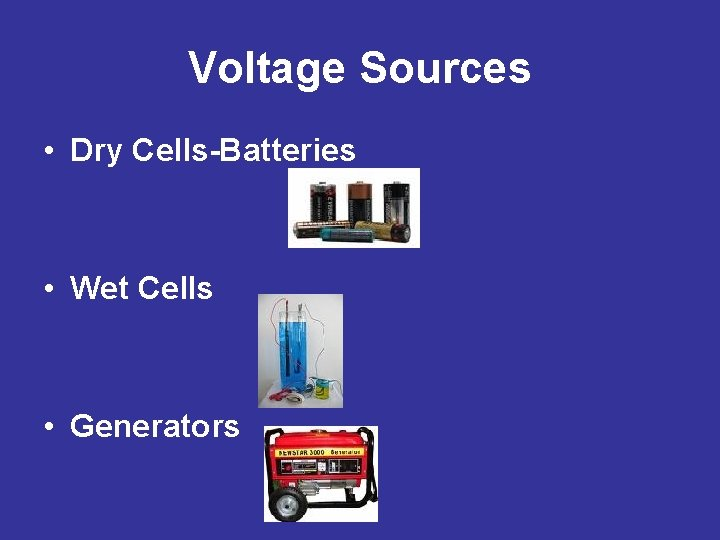Voltage Sources • Dry Cells-Batteries • Wet Cells • GeneratorsElectric Current • Direct Current Flows in 1 Direction Only • DC is produced when stored electrical energy is tapped • Batteries and Solar Cells have this electrical potential • Alternating Current changes direction periodically • AC is produced by a generatorPower • A charge moving in a circuit expends energy. • This might result in heating the circuit, or turning a motor. • The rate at which electrical energy is converted to another form, such as mechanical, heat or light, is called Electric Power.• Electric Power=Current x Voltage =ampere x volt watt = watt P = I x V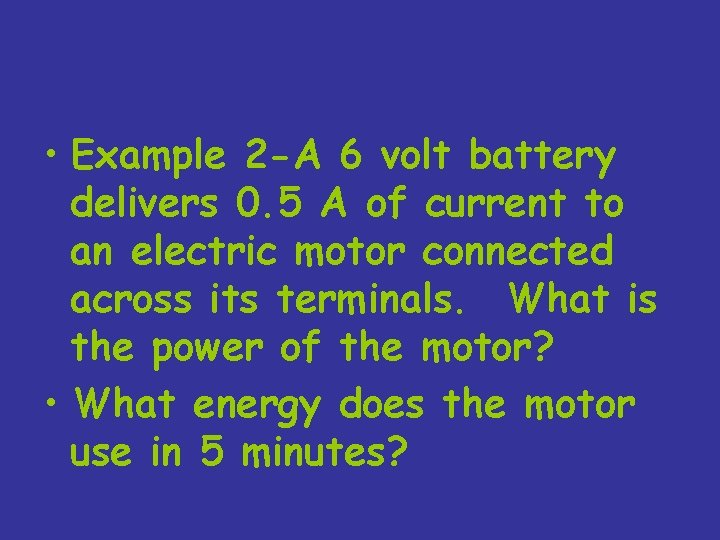• Example 2 -A 6 volt battery delivers 0. 5 A of current to an electric motor connected across its terminals. What is the power of the motor? • What energy does the motor use in 5 minutes?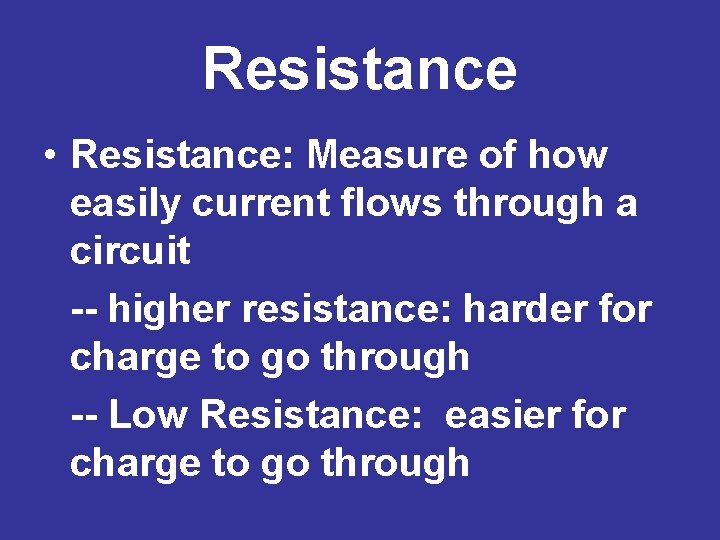Resistance • Resistance: Measure of how easily current flows through a circuit -- higher resistance: harder for charge to go through -- Low Resistance: easier for charge to go through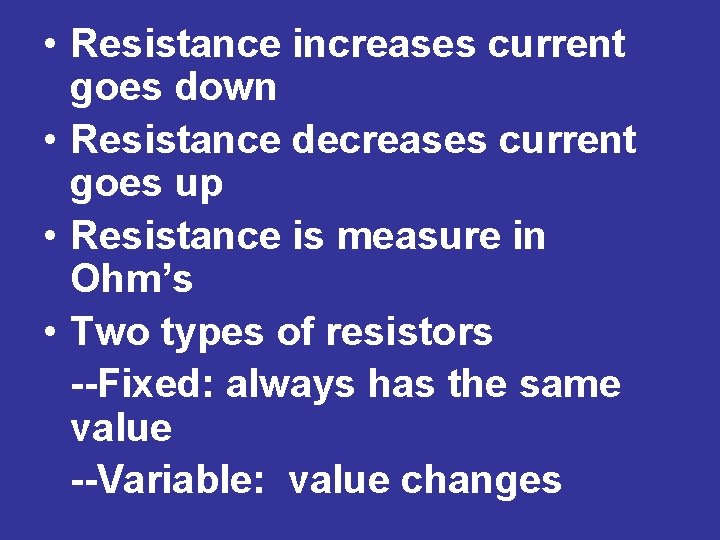• Resistance increases current goes down • Resistance decreases current goes up • Resistance is measure in Ohm’s • Two types of resistors --Fixed: always has the same value --Variable: value changes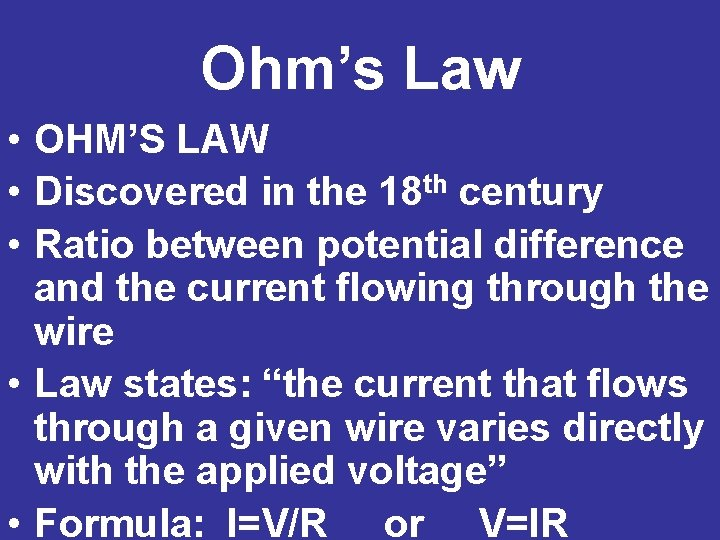Ohm’s Law • OHM’S LAW • Discovered in the 18 th century • Ratio between potential difference and the current flowing through the wire • Law states: “the current that flows through a given wire varies directly with the applied voltage” • Formula: I=V/R or V=IROhm’s Law-Current Flow • Symbol for ohm is (see board); 1 ohm is the resistance which permits a current of 1 A to flow between a voltage of 1 • To obtain a higher voltage, higher charge is needed; higher voltage=electric field to be more intense=more electrons to move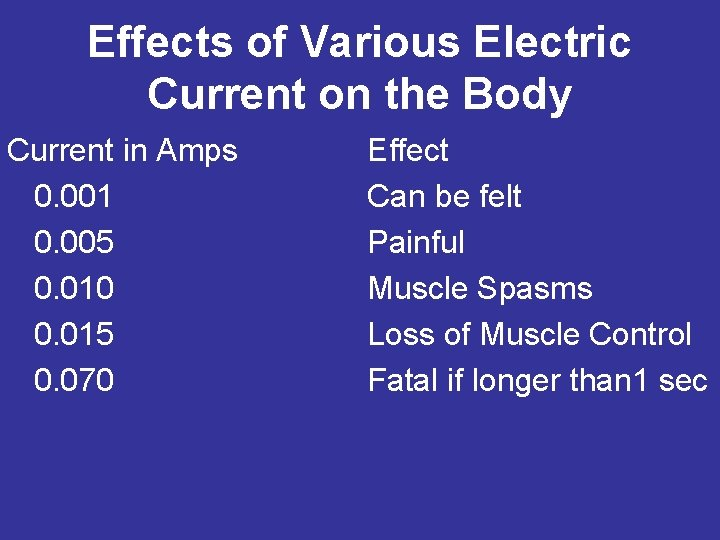Effects of Various Electric Current on the Body Current in Amps 0. 001 0. 005 0. 010 0. 015 0. 070 Effect Can be felt Painful Muscle Spasms Loss of Muscle Control Fatal if longer than 1 sec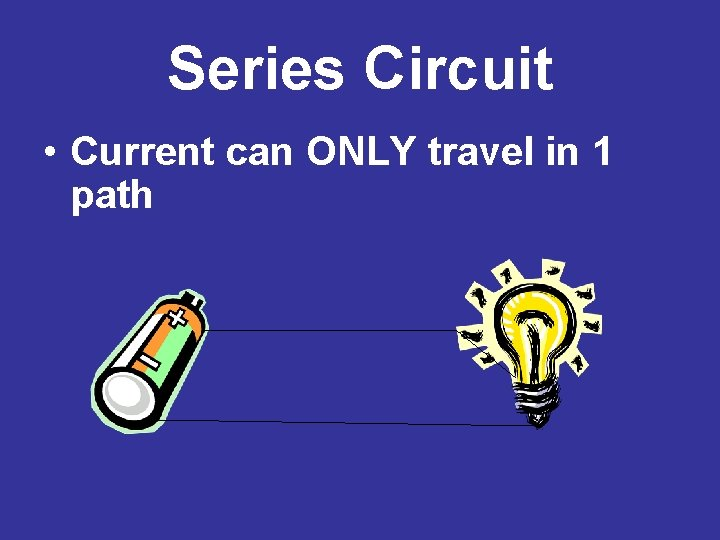Series Circuit • Current can ONLY travel in 1 pathSeries Circuit-math • Formula to find “total resistance” RT = R 1 + R 2 + etc To find the current flow: use Ohm’s Law The sum of the voltage is equal to the voltage of the entire circuit put together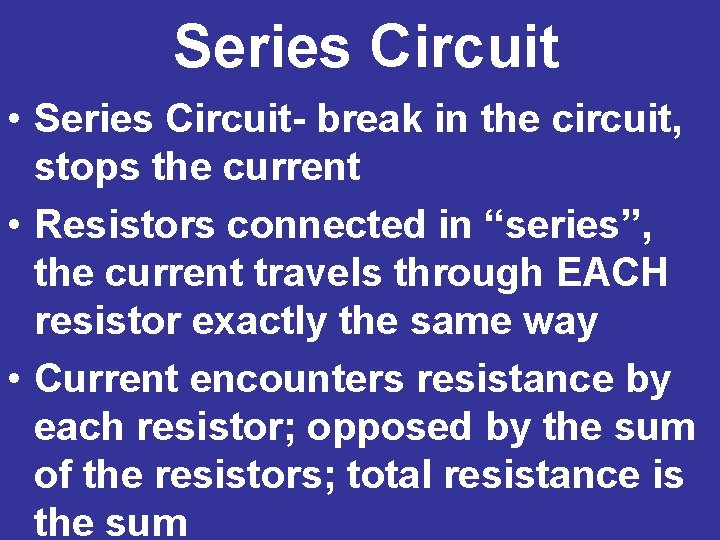Series Circuit • Series Circuit- break in the circuit, stops the current • Resistors connected in “series”, the current travels through EACH resistor exactly the same way • Current encounters resistance by each resistor; opposed by the sum of the resistors; total resistance is the sum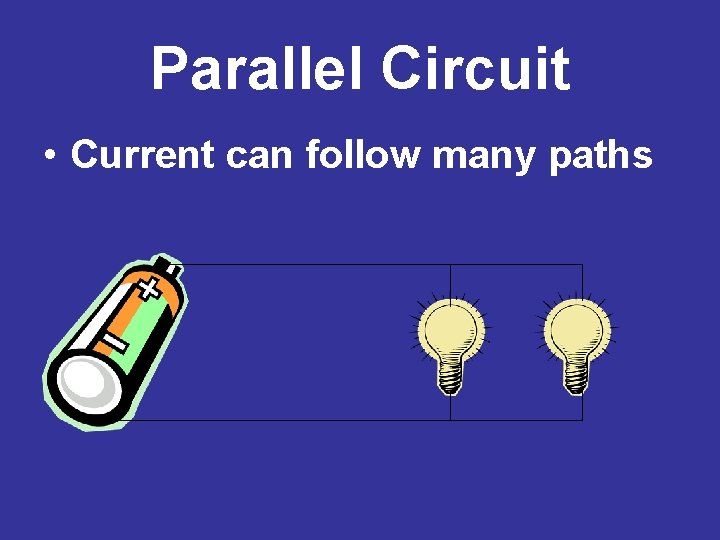Parallel Circuit • Current can follow many pathsParallel Circuit • Placing resistors in parallel always decreases the total resistance of the circuit! • Total resistance decreases because each new resistor has a “different” path for the electrons to flow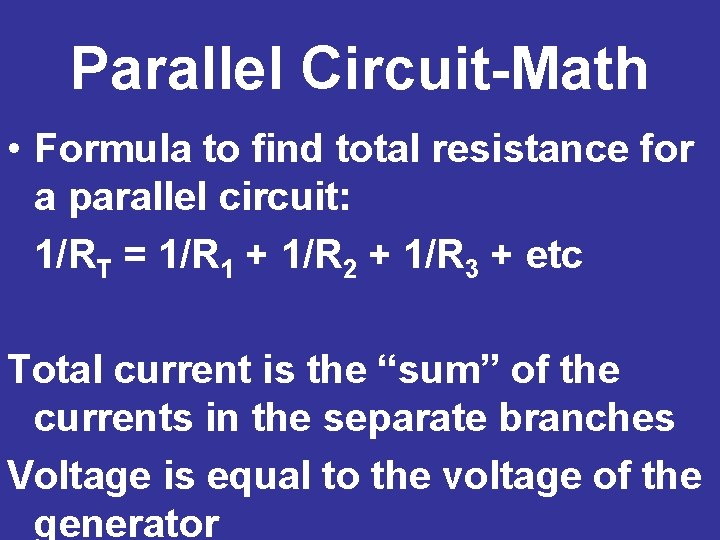Parallel Circuit-Math • Formula to find total resistance for a parallel circuit: 1/RT = 1/R 1 + 1/R 2 + 1/R 3 + etc Total current is the “sum” of the currents in the separate branches Voltage is equal to the voltage of the generator